# “CSelCalcCombineFilters_ExponentialBackoff” Calculator– Part II

At the end of my last post, “CSelCalcCombineFilters_ExponentialBackoff” Calculator I gave the following homework:

Homework assignment – add another member column to the mix that has a 0.100 selectivity (for example – county) and compare the estimates using a pre-2014 and 2014 CTP2 compatibility mode.

Here is the answer-key.  I’ll start off with adding a new column called “county” to the member table (and yes – county gets a little involved for some cities like NYC, but let’s just go with a uniform county association to city/state for now):

```ALTER TABLE [dbo].[member]
```

And then next I’ll revise my previous city/state update script to include county:

```UPDATE  [dbo].[member]
SET     [city] = 'Minneapolis',
[state_prov] = 'MN',
[county] = 'Hennepin'
WHERE   [member_no] % 10 = 0;

UPDATE  [dbo].[member]
SET     [city] = 'New York',
[state_prov] = 'NY',
[county]='Manhattan'
WHERE   [member_no] % 10 = 1;

UPDATE  [dbo].[member]
SET     [city] = 'Chicago',
[state_prov] = 'IL',
[county]='Cook'
WHERE   [member_no] % 10 = 2;

UPDATE  [dbo].[member]
SET     [city] = 'Houston',
[state_prov] = 'TX',
[county]='Harrisburg'
WHERE   [member_no] % 10 = 3;

UPDATE  [dbo].[member]
[state_prov] = 'PA',
WHERE   [member_no] % 10 = 4;

UPDATE  [dbo].[member]
SET     [city] = 'Phoenix',
[state_prov] = 'AZ',
[county]=''
WHERE   [member_no] % 10 = 5;

UPDATE  [dbo].[member]
SET     [city] = 'San Antonio',
[state_prov] = 'TX',
[county]='Bexar'
WHERE   [member_no] % 10 = 6;

UPDATE  [dbo].[member]
SET     [city] = 'San Diego',
[state_prov] = 'CA',
[county]='San Diego'
WHERE   [member_no] % 10 = 7;

UPDATE  [dbo].[member]
SET     [city] = 'Dallas',
[state_prov] = 'TX',
[county]='Dallas'
WHERE   [member_no] % 10 = 8;
GO
```

Now, what row estimate will we get for the member table pre-2014 if we execute the following query?

```SELECT  m.[lastname],
m.[firstname],
SUM(c.[charge_amt]) AS [Total_Charge_amt]
FROM    [dbo].[member] AS [m]
INNER JOIN [dbo].[charge] AS  ON
m.[member_no] = c.[member_no]
WHERE   [city] = 'Minneapolis' AND
[state_prov] = 'MN' AND
[county] = 'Hennepin'
GROUP BY m.[lastname],
m.[firstname]
OPTION (RECOMPILE);
GO
```

We see the following estimated rows versus actual (keeping in mind that there are no multi-column stats to help us out):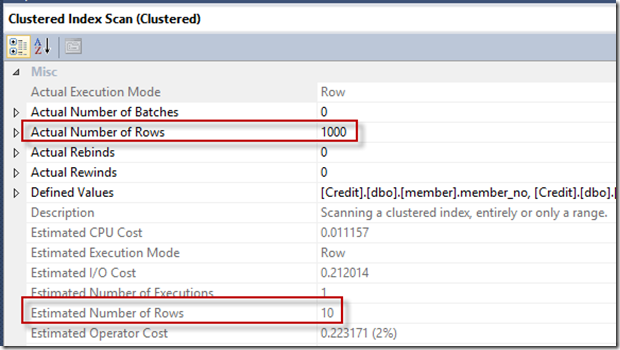Estimated number of rows is 10, but actual is 1000.

Given that we know the three predicates are correlated, but SQL Server thinks they are independent (no multi-column stats), we can see the calculation is as follows: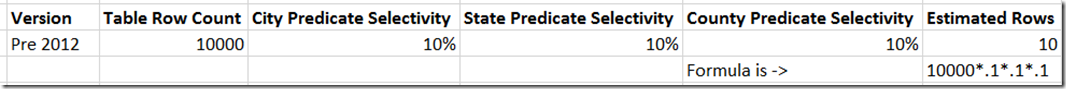Now if I switch to 2014 compatibility mode, I see the following estimated number of rows: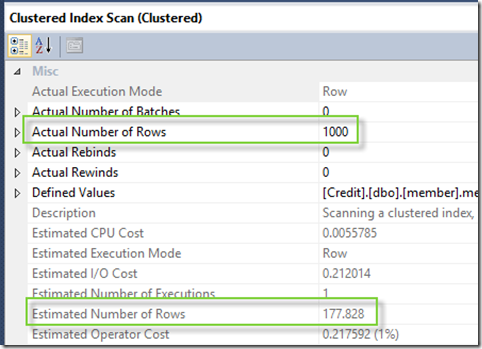And I see the following query_optimizer_estimate_cardinality event output we see the following for the 2014 version of the plan:

<CalculatorList>
<FilterCalculator CalculatorName=”CSelCalcCombineFilters_ExponentialBackoff” Conjunction=”true”>
<SubCalculator>
<FilterCalculator CalculatorName=”CSelCalcColumnInInterval” Selectivity=”0.100″ TableName=”[m]” ColumnName=”state_prov” StatId=”5″ />
<FilterCalculator CalculatorName=”CSelCalcColumnInInterval” Selectivity=”0.100″ TableName=”[m]” ColumnName=”county” StatId=”8″ />
<FilterCalculator CalculatorName=”CSelCalcColumnInInterval” Selectivity=”0.100″ TableName=”[m]” ColumnName=”city” StatId=”4″ />
</SubCalculator>
</FilterCalculator>
</CalculatorList>

So updating the formulas, how is the 177.828 derived?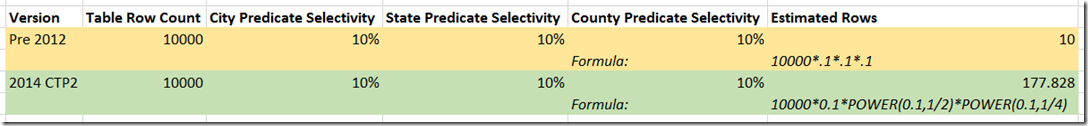So we see in 2014 CTP2 that instead of multiplying the selectivity of each predicate (again, assuming independence), the Exponential Backoff calculator is blunting the impact 10000*0.1*POWER(0.1,1/2)*POWER(0.1,1/4).

Still a skew – but less so.  177.828 estimated vs. 10.  And think it doesn’t really matter?

For this example on my test system, pre-2014 the Clustered Index Scan was serial and fed to a Distribute Streams exchange operator, and with the new estimate, the operator is parallel.

Before: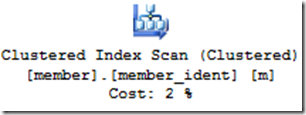After:Plenty more to explore in this area…

## Other articles

### New Course: “SQL Server: Common Query Tuning Problems and Solutions – Part 2″

Today, Pluralsight published my latest course, “SQL Server: Common Query Tuning Problems and Solutions – Part 2“.  Part two is 2 hours and 17 minutes long

Explore

### New Course: “SQL Server: Common Query Tuning Problems and Solutions – Part 1”

Pluralsight published my latest course, “SQL Server: Common Query Tuning Problems and Solutions – Part 1“.  Part 1 is 2 hours and 31 minutes and

Explore

### SQLIntersection Post-Conference Session

I am really happy to announce that Kimberly Tripp and I will be delivering a post-conference in November’s SQLIntersection conference at the MGM Grand. The

Explore

### Optimizing Your Query Plans with the SQL Server 2014 Cardinality Estimator

I’ve been working on writing a new Microsoft white paper since January covering the main changes made in the SQL Server 2014 Cardinality Estimator, and

Explore

### Finding Timeout-Prone Code with max_elapsed_time

Consider a scenario where your application timeout setting is 30 seconds and you’ve been asked to proactively start tuning workloads that are at risk of

Explore

### Deck from “Practical SQL Server Cardinality Estimation”

I uploaded the deck from my session for SQLSaturday #287 Madison 2014 and you can download it here. It was an excellent event!  Great organizers,

Explore

## Imagine feeling confident enough to handle whatever your database throws at you.

With training and consulting from SQLskills, you’ll be able to solve big problems, elevate your team’s capacity, and take control of your data career.